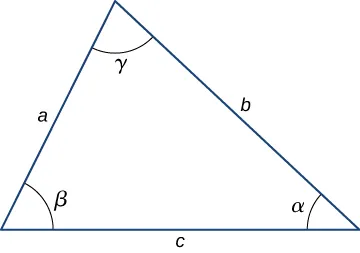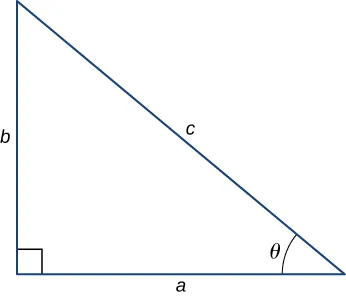University Physics Volume 2

# E | Mathematical Formulas

University Physics Volume 2E | Mathematical Formulas

If $ax2+bx+c=0,ax2+bx+c=0,$ then $x=−b±b2−4ac2ax=−b±b2−4ac2a$

Triangle of base $bb$ and height $hh$ Area $=12bh=12bh$
Circle of radius $rr$ Circumference $=2πr=2πr$ Area $=πr2=πr2$
Sphere of radius $rr$ Surface area $=4πr2=4πr2$ Volume $=43πr3=43πr3$
Cylinder of radius $rr$ and height $hh$ Area of curved surface $=2πrh=2πrh$ Volume $=πr2h=πr2h$
Table E1 Geometry

Trigonometry

Trigonometric Identities

1. $sinθ=1/cscθsinθ=1/cscθ$
2. $cosθ=1/secθcosθ=1/secθ$
3. $tanθ=1/cotθtanθ=1/cotθ$
4. $sin(900−θ)=cosθsin(900−θ)=cosθ$
5. $cos(900−θ)=sinθcos(900−θ)=sinθ$
6. $tan(900−θ)=cotθtan(900−θ)=cotθ$
7. $sin2θ+cos2θ=1sin2θ+cos2θ=1$
8. $sec2θ−tan2θ=1sec2θ−tan2θ=1$
9. $tanθ=sinθ/cosθtanθ=sinθ/cosθ$
10. $sin(α±β)=sinαcosβ±cosαsinβsin(α±β)=sinαcosβ±cosαsinβ$
11. $cos(α±β)=cosαcosβ∓sinαsinβcos(α±β)=cosαcosβ∓sinαsinβ$
12. $tan(α±β)=tanα±tanβ1∓tanαtanβtan(α±β)=tanα±tanβ1∓tanαtanβ$
13. $sin2θ=2sinθcosθsin2θ=2sinθcosθ$
14. $cos2θ=cos2θ−sin2θ=2cos2θ−1=1−2sin2θcos2θ=cos2θ−sin2θ=2cos2θ−1=1−2sin2θ$
15. $sinα+sinβ=2sin12(α+β)cos12(α−β)sinα+sinβ=2sin12(α+β)cos12(α−β)$
16. $cosα+cosβ=2cos12(α+β)cos12(α−β)cosα+cosβ=2cos12(α+β)cos12(α−β)$
17. $s=rθs=rθ$

Triangles

1. Law of sines: $asinα=bsinβ=csinγasinα=bsinβ=csinγ$
2. Law of cosines: $c2=a2+b2−2abcosγc2=a2+b2−2abcosγ$3. Pythagorean theorem: $a2+b2=c2a2+b2=c2$Series expansions

1. Binomial theorem: $(a+b)n=an+nan−1b+n(n−1)an−2b22!+n(n−1)(n−2)an−3b33!+···(a+b)n=an+nan−1b+n(n−1)an−2b22!+n(n−1)(n−2)an−3b33!+···$
2. $(1±x)n=1±nx1!+n(n−1)x22!±···(x2<1)(1±x)n=1±nx1!+n(n−1)x22!±···(x2<1)$
3. $(1±x)−n=1∓nx1!+n(n+1)x22!∓···(x2<1)(1±x)−n=1∓nx1!+n(n+1)x22!∓···(x2<1)$
4. $sinx=x−x33!+x55!−···sinx=x−x33!+x55!−···$
5. $cosx=1−x22!+x44!−···cosx=1−x22!+x44!−···$
6. $tanx=x+x33+2x515+···tanx=x+x33+2x515+···$
7. $ex=1+x+x22!+···ex=1+x+x22!+···$
8. $ln(1+x)=x−12x2+13x3−···(|x|<1)ln(1+x)=x−12x2+13x3−···(|x|<1)$

Derivatives

1. $ddx[af(x)]=addxf(x)ddx[af(x)]=addxf(x)$
2. $ddx[f(x)+g(x)]=ddxf(x)+ddxg(x)ddx[f(x)+g(x)]=ddxf(x)+ddxg(x)$
3. $ddx[f(x)g(x)]=f(x)ddxg(x)+g(x)ddxf(x)ddx[f(x)g(x)]=f(x)ddxg(x)+g(x)ddxf(x)$
4. $ddxf(u)=[dduf(u)]dudxddxf(u)=[dduf(u)]dudx$
5. $ddxxm=mxm−1ddxxm=mxm−1$
6. $ddxsinx=cosxddxsinx=cosx$
7. $ddxcosx=−sinxddxcosx=−sinx$
8. $ddxtanx=sec2xddxtanx=sec2x$
9. $ddxcotx=−csc2xddxcotx=−csc2x$
10. $ddxsecx=tanxsecxddxsecx=tanxsecx$
11. $ddxcscx=−cotxcscxddxcscx=−cotxcscx$
12. $ddxex=exddxex=ex$
13. $ddxlnx=1xddxlnx=1x$
14. $ddxsin−1x=11−x2ddxsin−1x=11−x2$
15. $ddxcos−1x=−11−x2ddxcos−1x=−11−x2$
16. $ddxtan−1x=11+x2ddxtan−1x=11+x2$

Integrals

1. $∫af(x)dx=a∫f(x)dx∫af(x)dx=a∫f(x)dx$
2. $∫[f(x)+g(x)]dx=∫f(x)dx+∫g(x)dx∫[f(x)+g(x)]dx=∫f(x)dx+∫g(x)dx$
3. $∫xmdx=xm+1m+1(m≠−1)=lnx(m=−1)∫xmdx=xm+1m+1(m≠−1)=lnx(m=−1)$
4. $∫sinxdx=−cosx∫sinxdx=−cosx$
5. $∫cosxdx=sinx∫cosxdx=sinx$
6. $∫tanxdx=ln|secx|∫tanxdx=ln|secx|$
7. $∫sin2axdx=x2−sin2ax4a∫sin2axdx=x2−sin2ax4a$
8. $∫cos2axdx=x2+sin2ax4a∫cos2axdx=x2+sin2ax4a$
9. $∫sinaxcosaxdx=−cos2ax4a∫sinaxcosaxdx=−cos2ax4a$
10. $∫eaxdx=1aeax∫eaxdx=1aeax$
11. $∫xeaxdx=eaxa2(ax−1)∫xeaxdx=eaxa2(ax−1)$
12. $∫lnaxdx=xlnax−x∫lnaxdx=xlnax−x$
13. $∫dxa2+x2=1atan−1xa∫dxa2+x2=1atan−1xa$
14. $∫dxa2−x2=12aln|x+ax−a|∫dxa2−x2=12aln|x+ax−a|$
15. $∫dxa2+x2=sinh−1xa∫dxa2+x2=sinh−1xa$
16. $∫dxa2−x2=sin−1xa∫dxa2−x2=sin−1xa$
17. $∫a2+x2dx=x2a2+x2+a22sinh−1xa∫a2+x2dx=x2a2+x2+a22sinh−1xa$
18. $∫a2−x2dx=x2a2−x2+a22sin−1xa∫a2−x2dx=x2a2−x2+a22sin−1xa$
Order a print copy

As an Amazon Associate we earn from qualifying purchases.